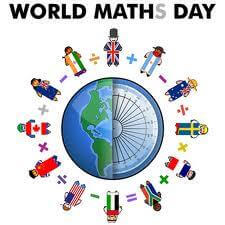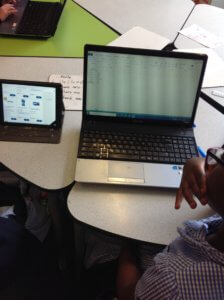# Year 4 Number Day ActivitiesFor number Day, year 4 created their own shopping list and used Excel to find how much money they would be spending. Then searched for their items on Tesco to find out how much they all were. They typed the cost of each item into a spreadsheet and then used a formula to calculate the total cost (addition). They used another formula to calculate the total cost of items if they had more than one(multiplication). They discussed why we needed to use the different operations for different parts.